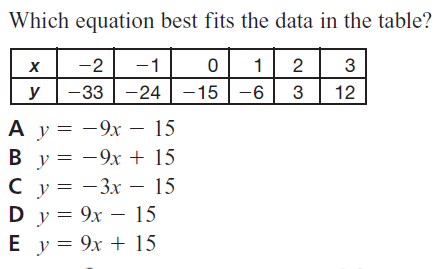GeoB Enrichment 4
Email me when this is complete so I know to check it
A piece of string that is 132 inches long is cut into 3 pieces. The second piece of string is twice as long as the first piece. The third piece of string is three times as long as the first piece. Find the length of the longest piece of string. *
1 point
Fractions *
1 point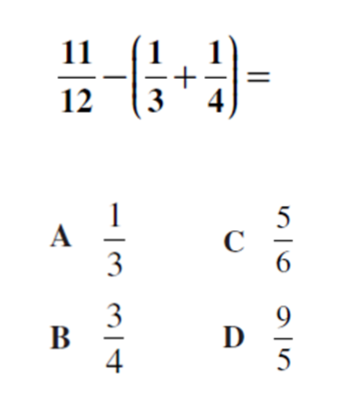At the grocery store, Jerry spent \$24.75 for 3 pounds of chicken and 3 pounds of cheese.The cheese cost \$4.50 per pound. What was the cost of the chicken per pound? *
1 point
Fractions *
1 pointName *
solve *
1 pointThe length of a rectangle is 50 cm. longer than the width. If the perimeter of the rectangle is 220 cm., find the width and length. *
1 point
Find the indicated angle *
1 point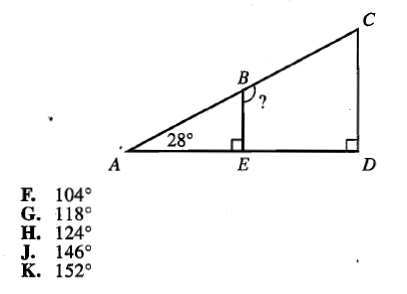Quandalyn types 5 words in 15 seconds. How many words can she type in an hour at the same rate? *
1 point
polynomials *
1 point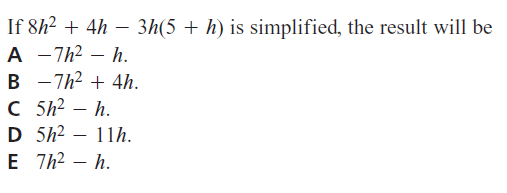Volume *
1 point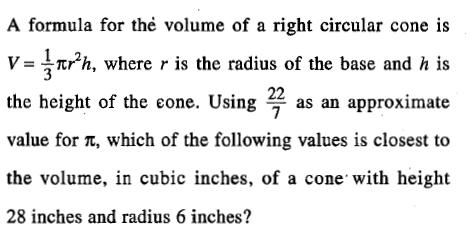Word problems *
1 point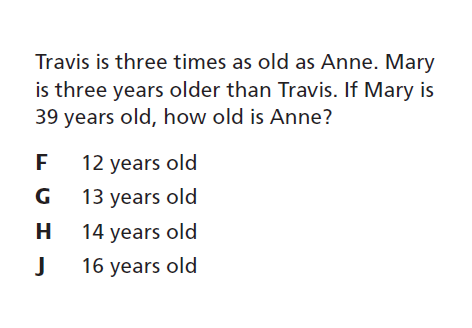period *
Linear equations *
1 point